Ex 7.2

Chapter 7 Class 10 Coordinate Geometry
Serial order wise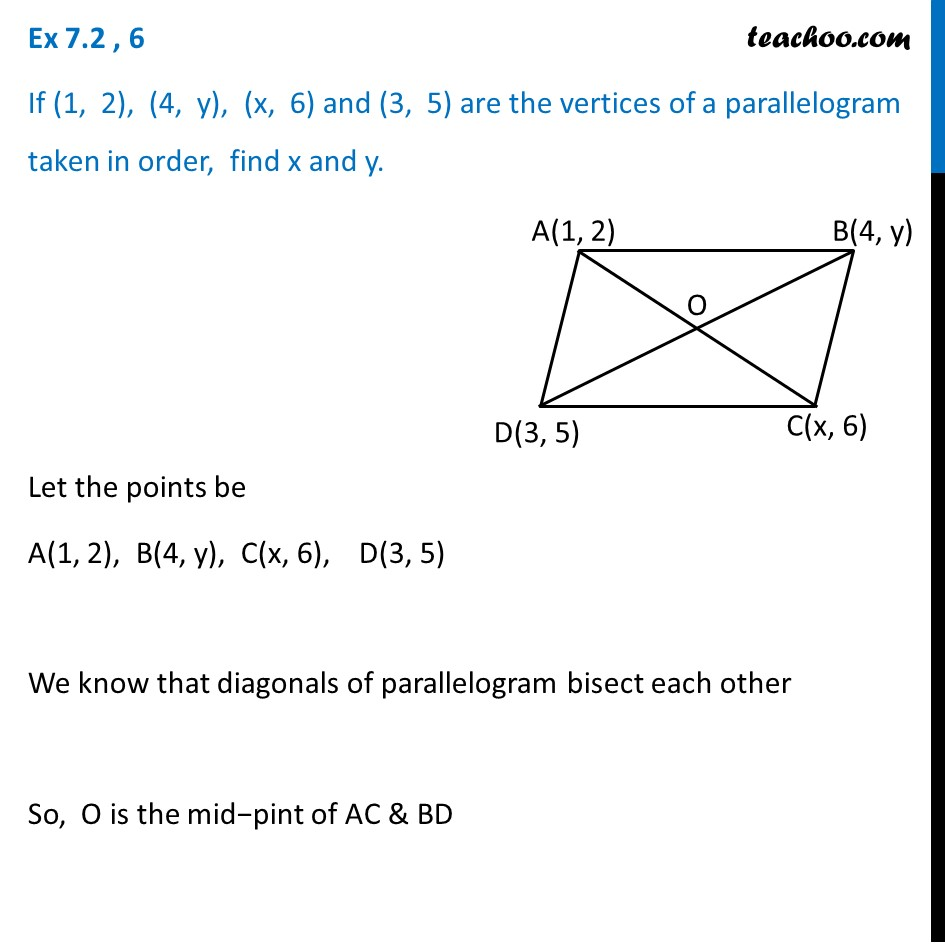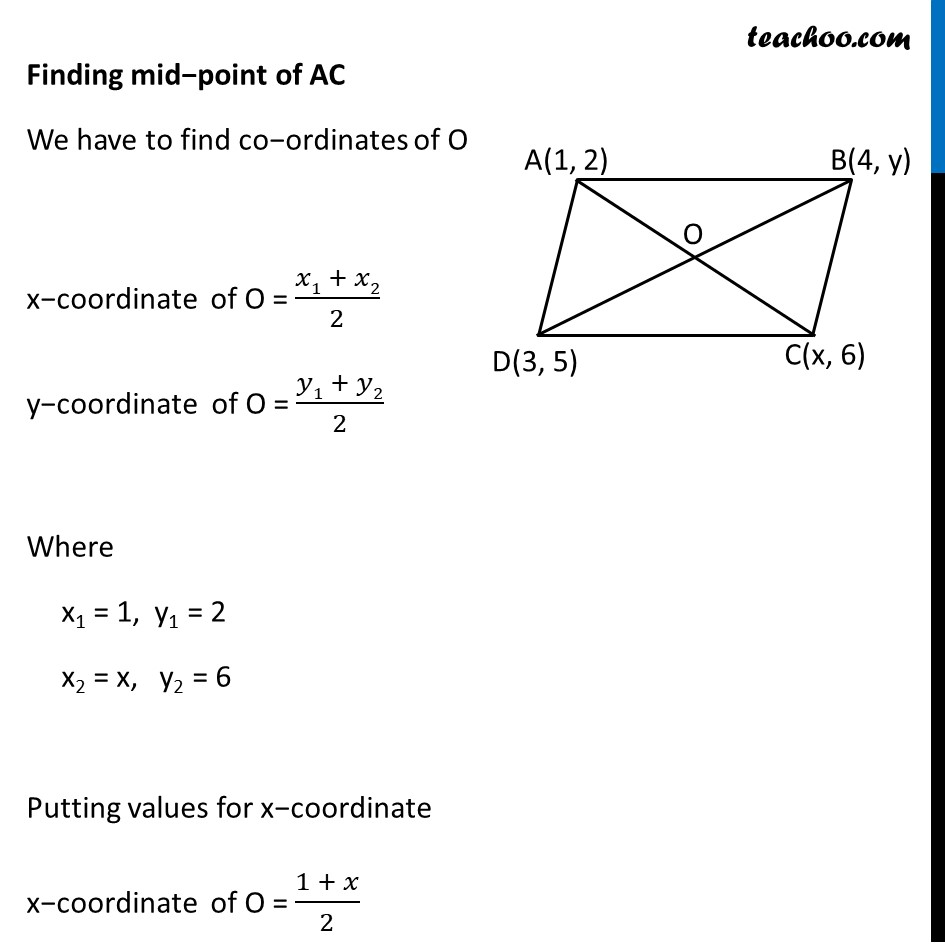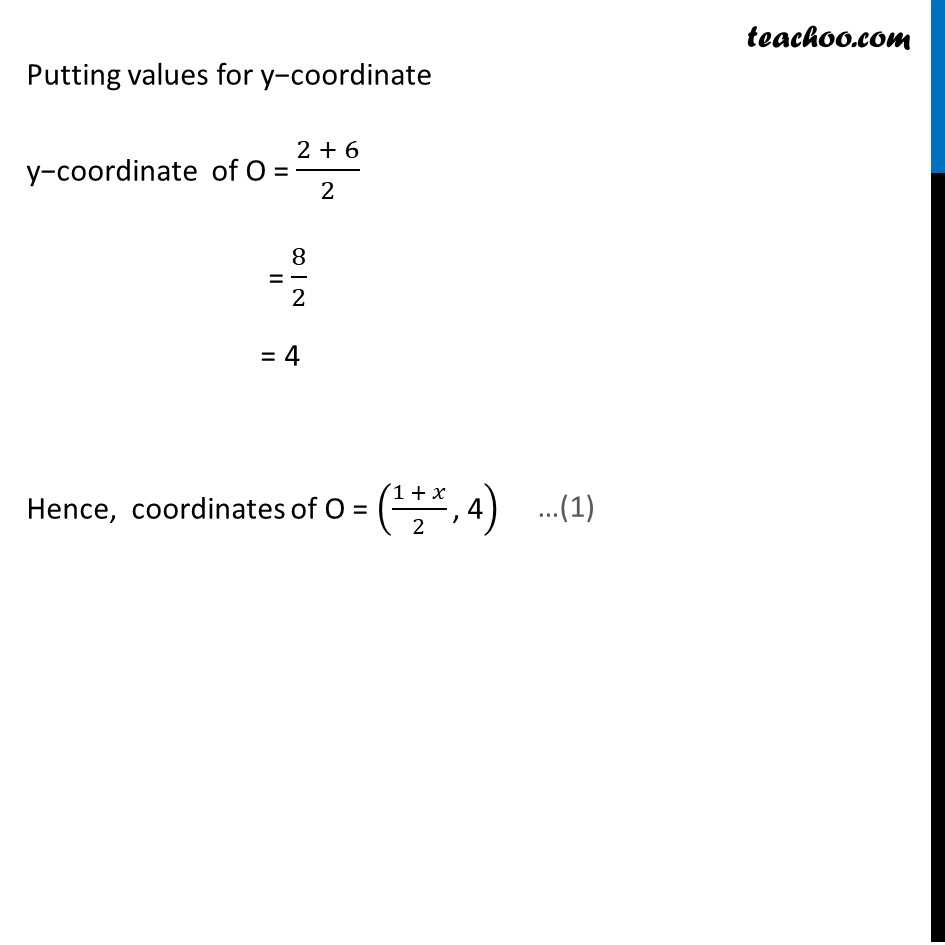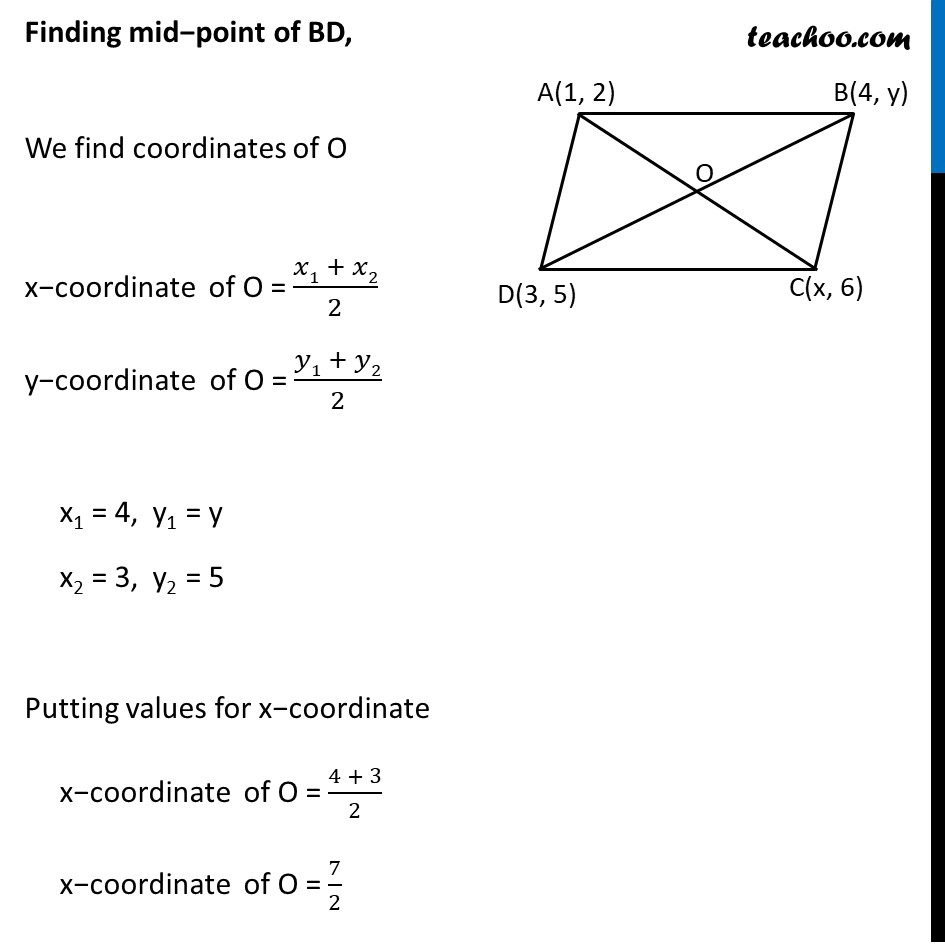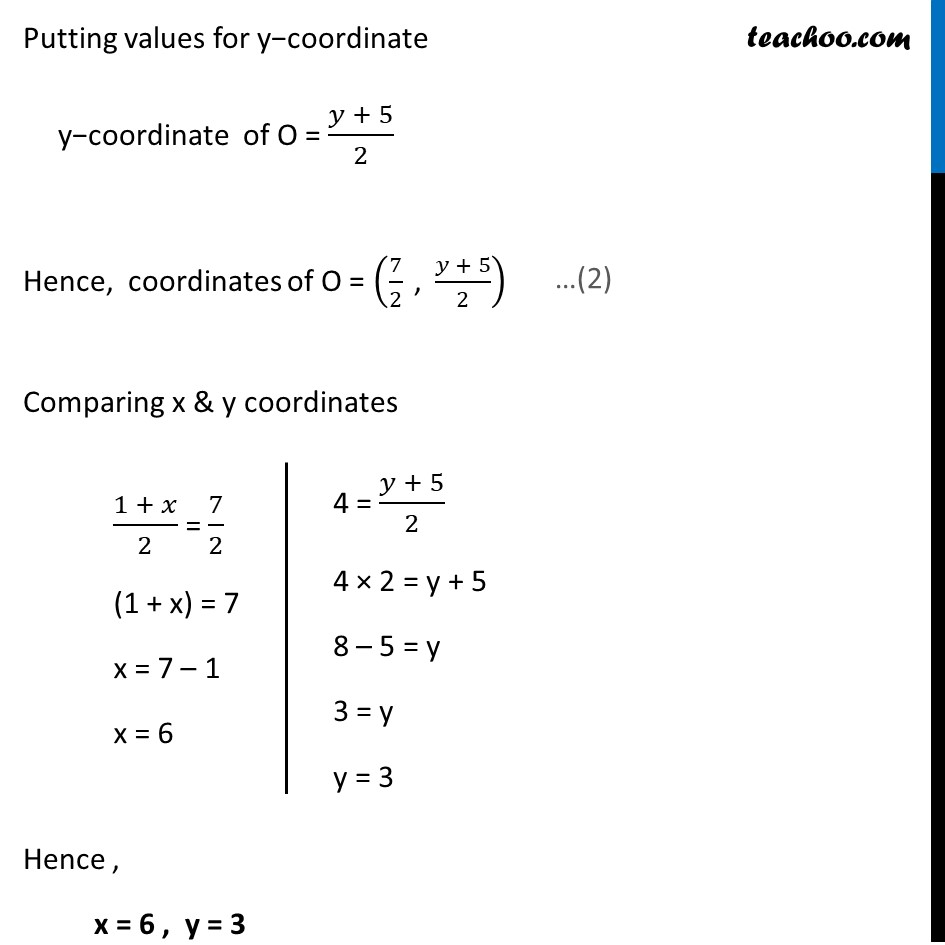Learn in your speed, with individual attention - Teachoo Maths 1-on-1 Class

### Transcript

Ex 7.2 , 6 If (1, 2), (4, y), (x, 6) and (3, 5) are the vertices of a parallelogram taken in order, find x and y. Let the points be A(1, 2), B(4, y), C(x, 6), D(3, 5) We know that diagonals of parallelogram bisect each other So, O is the mid−pint of AC & BD Finding mid−point of AC We have to find co−ordinates of O x−coordinate of O = (𝑥1 + 𝑥2)/2 y−coordinate of O = (𝑦1 + 𝑦2)/2 Where x1 = 1, y1 = 2 x2 = x, y2 = 6 Putting values for x−coordinate x−coordinate of O = (1 + 𝑥)/2 Putting values for y−coordinate y−coordinate of O = (2 + 6)/2 = 8/2 = 4 Hence, coordinates of O = ((1 + 𝑥)/2 ", 4" ) Finding mid−point of BD, We find coordinates of O x−coordinate of O = (𝑥1 + 𝑥2)/2 y−coordinate of O = (𝑦1 + 𝑦2)/2 x1 = 4, y1 = y x2 = 3, y2 = 5 Putting values for x−coordinate x−coordinate of O = (4 + 3)/2 x−coordinate of O = 7/2 Putting values for y−coordinate y−coordinate of O = (𝑦 + 5)/2 Hence, coordinates of O = (7/2 " , " (𝑦 + 5)/2) Comparing x & y coordinates Hence , x = 6 , y = 3 (1 + 𝑥)/2 = 7/2 (1 + x) = 7 x = 7 – 1 x = 6 4 = (𝑦 + 5)/2 4 × 2 = y + 5 8 – 5 = y 3 = y y = 3